Friday, 30 March 2018 08:39

### Add a Node to SmartArt in PowerPoint in C#

With the help of Spire.Presentation, we can easily add SmartArt shape to the presentation slides. We can add a new node to the existing SmartArt shape to presentation slides and the following code example demonstrates the same.

Step 1: Create a new PowerPoint document and load the sample document from file.

```Presentation presentation = new Presentation();
```

Step 2: Get the SmartArt from the presentation slide.

```ISmartArt sa = presentation.Slides.Shapes as ISmartArt;
```

```ISmartArtNode node = sa.Nodes.AddNode();
```

Step 4: Add text and set the text style for the node.

```node.TextFrame.Text = "NewStep";
node.TextFrame.TextRange.Fill.FillType = Spire.Presentation.Drawing.FillFormatType.Solid;
node.TextFrame.TextRange.Fill.SolidColor.KnownColor = KnownColors.HotPink;
```

Step 5: Save the document to file.

```presentation.SaveToFile("AddNode.pptx", FileFormat.Pptx2010);
```

Effective screenshot after adding a new node: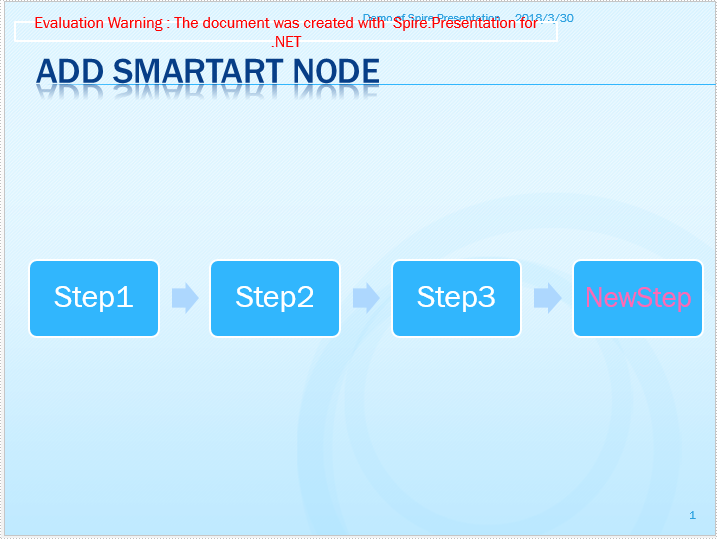Full codes of adding a node to SmartArt:

```Presentation presentation = new Presentation();

ISmartArt sa = presentation.Slides.Shapes as ISmartArt;

node.TextFrame.Text = "NewStep";
node.TextFrame.TextRange.Fill.FillType = Spire.Presentation.Drawing.FillFormatType.Solid;
node.TextFrame.TextRange.Fill.SolidColor.KnownColor = KnownColors.HotPink;

```
Wednesday, 28 March 2018 08:58

### Delete Blank Rows and Columns in Excel in C#

This article demonstrates how to delete blank rows and columns in an Excel worksheet using Spire.XLS and C#.

Example Excel file: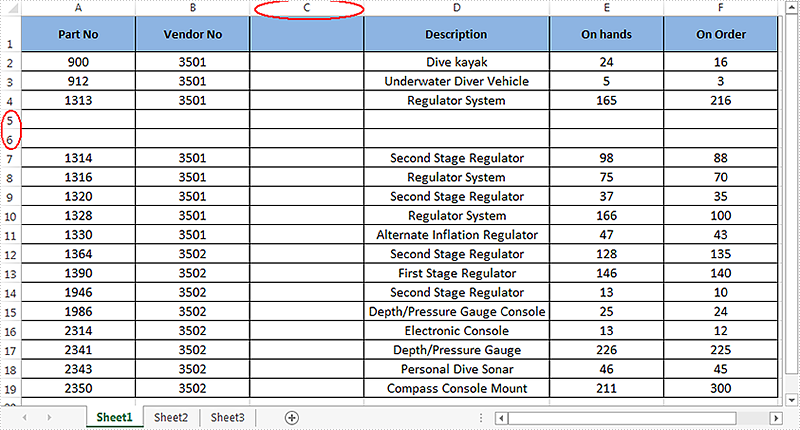Detail steps:

Step 1: Instantiate a Workbook object and load the Excel file.

```Workbook workbook = new Workbook();
```

Step 2: Get the first worksheet.

```Worksheet sheet = workbook.Worksheets;
```

Step 3: Delete blank rows from the worksheet.

```for (int i = sheet.Rows.Count() - 1; i >= 0; i--)
{
if (sheet.Rows[i].IsBlank)
{
sheet.DeleteRow(i+1);
}
}
```

Step 4: Delete blank columns from the worksheet.

```for (int j = sheet.Columns.Count() - 1; j >= 0; j--)
{
if (sheet.Columns[j].IsBlank)
{
sheet.DeleteColumn(j+1);
}
}
```

Step 5: Save the file.

```workbook.SaveToFile("DeleteBlankRowsAndColumns.xlsx", ExcelVersion.Version2013);
```

Output: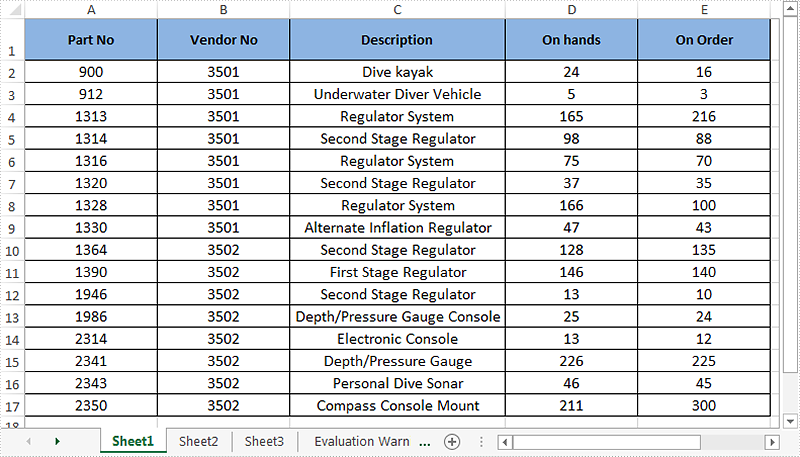Full code:

```using System;
using System.Collections.Generic;
using System.Linq;
using System.Text;
using Spire.Xls;

namespace Delete_Blank_Rows_and_Columns_in_Excel
{
class Program
{
static void Main(string[] args)
{
//Instantiate a Workbook object
Workbook workbook = new Workbook();

//Get the first worksheet
Worksheet sheet = workbook.Worksheets;

//Delete blanks rows
for (int i = sheet.Rows.Count() - 1; i >= 0; i--)
{
if (sheet.Rows[i].IsBlank)
{
sheet.DeleteRow(i+1); //Index parameter in DeleteRow method starts from 1
}
}

//Delete blank columns
for (int j = sheet.Columns.Count() - 1; j >= 0; j--)
{
if (sheet.Columns[j].IsBlank)
{
sheet.DeleteColumn(j+1); //Index parameter in DeleteColumn method starts from 1
}
}

//Save the file
workbook.SaveToFile("DeleteBlankRowsAndColumns.xlsx", ExcelVersion.Version2013);
}
}
}
```
Monday, 26 March 2018 08:29

### Add a Cover Image when Converting Word to EPUB in C#, VB.NET

We already have the documentation introducing how to convert Word to EPUB. However, you may want to add a cover image to EPUB when creating an EPUB book from a Word document. The following code snippets will demonstrate the same.

Step 1: Create a Document instance and load a sample Word file.

```Document doc = new Document();
```

Step 2: Load a picture to DocPicture object.

```DocPicture picture = new DocPicture(doc);
```

Step 3: Add the picture to EPUB as cover image when creating EPUB from the Word document.

```doc.SaveToEpub("output.epub", picture);
```

Output: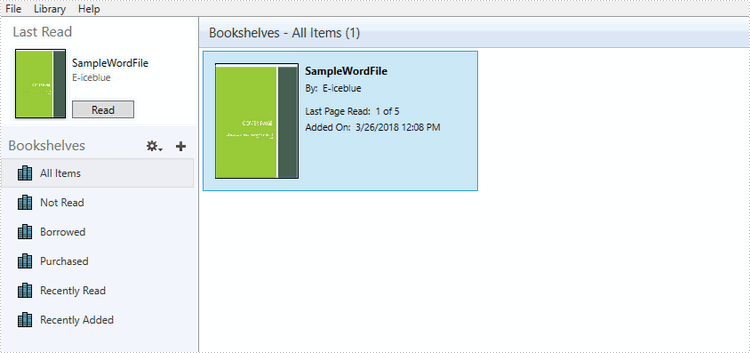Full Code:

[C#]
```Document doc = new Document();

DocPicture picture = new DocPicture(doc);
doc.SaveToEpub("output.epub", picture);
```
[VB.NET]
```Document doc  =  New Document()

Dim picture As DocPicture =  New DocPicture(doc)
doc.SaveToEpub("output.epub", picture)
```
Thursday, 22 March 2018 08:29

### Create 100% stacked bar chart in PowerPoint in C#

A 100% stacked bar chart is a chart type designed to show the relative percentage of multiple data series in stacked bars, where the total of each stacked bar always equals 100%. This article will demonstrate how to use Spire.Presentation to create 100% stacked bar in PowerPoint in C#.

Step 1: Create a Presentation object.

```Presentation presentation = new Presentation();
```

Step 2: Add a "Bar100PercentStacked" chart to the first slide.

```presentation.SlideSize.Type = SlideSizeType.Screen16x9;
SizeF slidesize = presentation.SlideSize.Size;
var slide = presentation.Slides;

RectangleF rect = new RectangleF(20, 20, slidesize.Width - 40, slidesize.Height - 40);
IChart chart = slide.Shapes.AppendChart(Spire.Presentation.Charts.ChartType.Bar100PercentStacked, rect);
```

Step 3: Write data to the chart data.

```string[] columnlabels = { "Series 1", "Series 2", "Series 3" };

// Insert the column labels
String[] cols = columnlabels.ToArray();
for (Int32 c = 0; c < cols.Count(); ++c)
chart.ChartData[0, c + 1].Text = cols[c];

string[] rowlabels = { "Category 1", "Category 2", "Category 3" };

// Insert the row labels
String[] rows = rowlabels.ToArray();
for (Int32 r = 0; r < rows.Count(); ++r)
chart.ChartData[r + 1, 0].Text = rows[r];

double[,] values = new double[3, 3] { { 20.83233, 10.34323, -10.354667 }, { 10.23456, -12.23456, 23.34456 }, { 12.34345, -23.34343, -13.23232 }};

// Insert the values
double value = 0.0;
for (Int32 r = 0; r < rows.Count(); ++r)
{
for (Int32 c = 0; c < cols.Count(); ++c)
{
value = Math.Round(values[r, c], 2);
chart.ChartData[r + 1, c + 1].Value = value;
}
}

chart.Series.SeriesLabel = chart.ChartData[0, 1, 0, columnlabels.Count()];
chart.Categories.CategoryLabels = chart.ChartData[1, 0, rowlabels.Count(), 0];

chart.PrimaryCategoryAxis.Position = AxisPositionType.Left;
chart.SecondaryCategoryAxis.Position = AxisPositionType.Left;
chart.PrimaryCategoryAxis.TickLabelPosition = TickLabelPositionType.TickLabelPositionLow;
```

Step 4: Set the data, font and format for the series of each column.

```for (Int32 c = 0; c < cols.Count(); ++c)
{
chart.Series[c].Values = chart.ChartData[1, c + 1, rowlabels.Count(), c + 1];
chart.Series[c].Fill.FillType = FillFormatType.Solid;
chart.Series[c].InvertIfNegative = false;

for (Int32 r = 0; r < rows.Count(); ++r)
{
label.LabelValueVisible = true;
chart.Series[c].DataLabels[r].HasDataSource = false;
chart.Series[c].DataLabels[r].NumberFormat = "0#\\%";
chart.Series[c].DataLabels.TextProperties.Paragraphs.DefaultCharacterProperties.FontHeight = 12;
}
}
chart.Series.Fill.SolidColor.Color = Color.YellowGreen;
chart.Series.Fill.SolidColor.Color = Color.Red;
chart.Series.Fill.SolidColor.Color = Color.Green;

TextFont font = new TextFont("Tw Cen MT");
```

Step 5: Set the font and size for chartlegend.

```for (int k = 0; k < chart.ChartLegend.EntryTextProperties.Length; k++)
{
chart.ChartLegend.EntryTextProperties[k].LatinFont = font;
chart.ChartLegend.EntryTextProperties[k].FontHeight = 20;
}
```

Step 6: Save the document to file.

```presentation.SaveToFile("Sample.pptx", FileFormat.Pptx2010);
```

Effective screenshot of 100% stacked bar chart: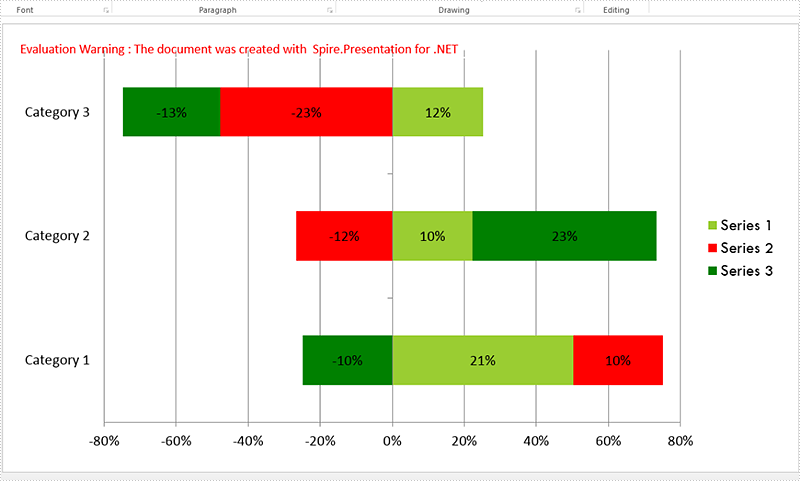Full codes:

```Presentation presentation = new Presentation();
presentation.SlideSize.Type = SlideSizeType.Screen16x9;
SizeF slidesize = presentation.SlideSize.Size;

var slide = presentation.Slides;

RectangleF rect = new RectangleF(20, 20, slidesize.Width - 40, slidesize.Height - 40);
IChart chart = slide.Shapes.AppendChart(Spire.Presentation.Charts.ChartType.Bar100PercentStacked, rect);

string[] columnlabels = { "Series 1", "Series 2", "Series 3" };

String[] cols = columnlabels.ToArray();
for (Int32 c = 0; c < cols.Count(); ++c)
chart.ChartData[0, c + 1].Text = cols[c];

string[] rowlabels = { "Category 1", "Category 2", "Category 3" };

String[] rows = rowlabels.ToArray();
for (Int32 r = 0; r < rows.Count(); ++r)
chart.ChartData[r + 1, 0].Text = rows[r];

double[,] values = new double[3, 3] { { 20.83233, 10.34323, -10.354667 }, { 10.23456, -12.23456, 23.34456 }, { 12.34345, -23.34343, -13.23232 }};

double value = 0.0;
for (Int32 r = 0; r < rows.Count(); ++r)
{
for (Int32 c = 0; c < cols.Count(); ++c)
{
value = Math.Round(values[r, c], 2);
chart.ChartData[r + 1, c + 1].Value = value;
}
}

chart.Series.SeriesLabel = chart.ChartData[0, 1, 0, columnlabels.Count()];
chart.Categories.CategoryLabels = chart.ChartData[1, 0, rowlabels.Count(), 0];

chart.PrimaryCategoryAxis.Position = AxisPositionType.Left;
chart.SecondaryCategoryAxis.Position = AxisPositionType.Left;
chart.PrimaryCategoryAxis.TickLabelPosition = TickLabelPositionType.TickLabelPositionLow;

for (Int32 c = 0; c < cols.Count(); ++c)
{
chart.Series[c].Values = chart.ChartData[1, c + 1, rowlabels.Count(), c + 1];
chart.Series[c].Fill.FillType = FillFormatType.Solid;
chart.Series[c].InvertIfNegative = false;

for (Int32 r = 0; r < rows.Count(); ++r)
{
label.LabelValueVisible = true;
chart.Series[c].DataLabels[r].HasDataSource = false;
chart.Series[c].DataLabels[r].NumberFormat = "0#\\%";
chart.Series[c].DataLabels.TextProperties.Paragraphs.DefaultCharacterProperties.FontHeight = 12;
}
}
chart.Series.Fill.SolidColor.Color = Color.YellowGreen;
chart.Series.Fill.SolidColor.Color = Color.Red;
chart.Series.Fill.SolidColor.Color = Color.Green;

TextFont font = new TextFont("Tw Cen MT");

for (int k = 0; k < chart.ChartLegend.EntryTextProperties.Length; k++)
{
chart.ChartLegend.EntryTextProperties[k].LatinFont = font;
chart.ChartLegend.EntryTextProperties[k].FontHeight = 20;
}

presentation.SaveToFile("Sample.pptx", FileFormat.Pptx2010);
```
Tuesday, 20 March 2018 09:35

### Print a PDF in Greyscale in C#

Below is the example PDF file we used for demonstration: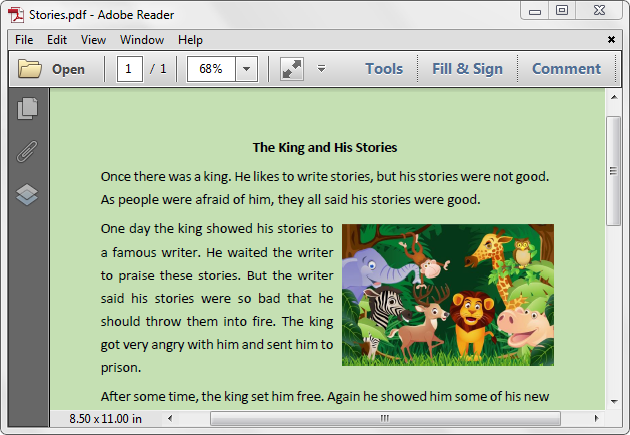Detail steps:

Step 1: Create a PdfDocument instance and load the PDF file.

```PdfDocument pdf = new PdfDocument();
```

Step 2: Set the PdfPrintSettings.Color property to false.

```pdf.PrintSettings.Color = false;
```

Step 3: Print the document.

```pdf.Print();
```

Screenshot after printing to xps: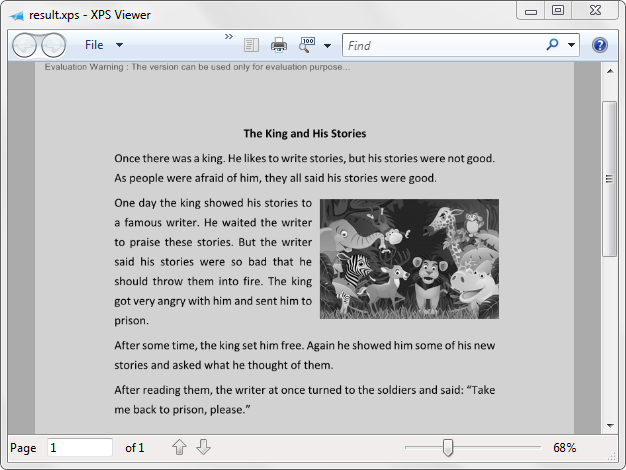Full code:

```using Spire.Pdf;

namespace Print_PDF_in_Black_and_White
{
class Program
{
static void Main(string[] args)
{
PdfDocument pdf = new PdfDocument();
pdf.PrintSettings.Color = false;
pdf.Print();
}
}
}
```
Monday, 19 March 2018 05:57

### Apply Shadow Effect to Shape in PowerPoint in C#

Shadows make your shapes or pictures especially with transparent background pop out of your slide. They make flat 2 dimensional graphics look like 3 dimensional graphics. This article will show you how we can apply shadow effects to shapes and pictures in PowerPoint using Spire.Presentation.

```//Create a Presentation object and get the first slide.
Presentation ppt = new Presentation();
ISlide slide = ppt.Slides;

RectangleF rect = new RectangleF(30, 80, 300, 120);
IAutoShape shape = slide.Shapes.AppendShape(ShapeType.Rectangle, rect);
shape.Fill.FillType = FillFormatType.Solid;
shape.Fill.SolidColor.Color = Color.LightBlue;
shape.Line.FillType = FillFormatType.None;
shape.TextFrame.Text = "This demo shows how to apply shadow effect to shape.";
shape.TextFrame.TextRange.Fill.FillType = FillFormatType.Solid;
shape.TextFrame.TextRange.Fill.SolidColor.Color = Color.Black;

//Apply the shadow effect to shape.

//Save to file.
```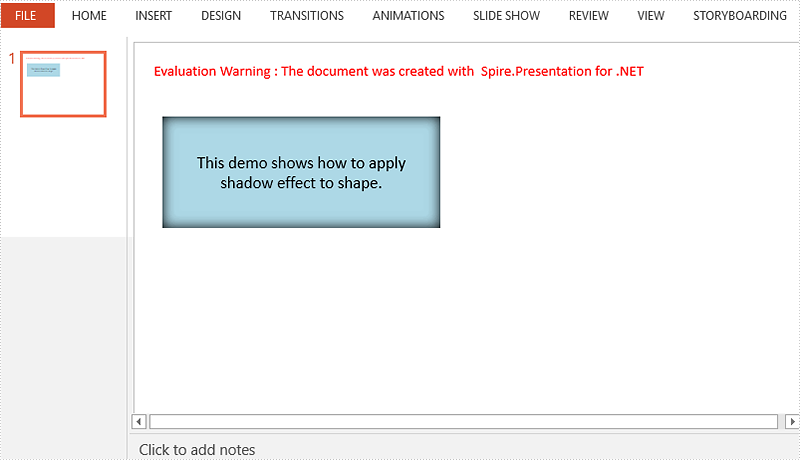```//Create a Presentation object and get the first slide.
Presentation ppt = new Presentation();
ISlide slide = ppt.Slides;

//Get the picture path.
string imagePath = "dinosaur.png";
Image image = Image.FromFile(imagePath);
float width = (float)image.Width / 3;
float height = (float)image.Height / 3;

//Add a shape to slide and fill the shape with picture.
RectangleF rect = new RectangleF(80, 80, width, height);
IAutoShape shape = slide.Shapes.AppendShape(ShapeType.Rectangle, rect);
shape.Fill.FillType = FillFormatType.Picture;
shape.Fill.PictureFill.Picture.Url = imagePath;
shape.Fill.PictureFill.FillType = PictureFillType.Stretch;
shape.Line.FillType = FillFormatType.None;

//Apply the shadow effect to shape.

//Save to file.
```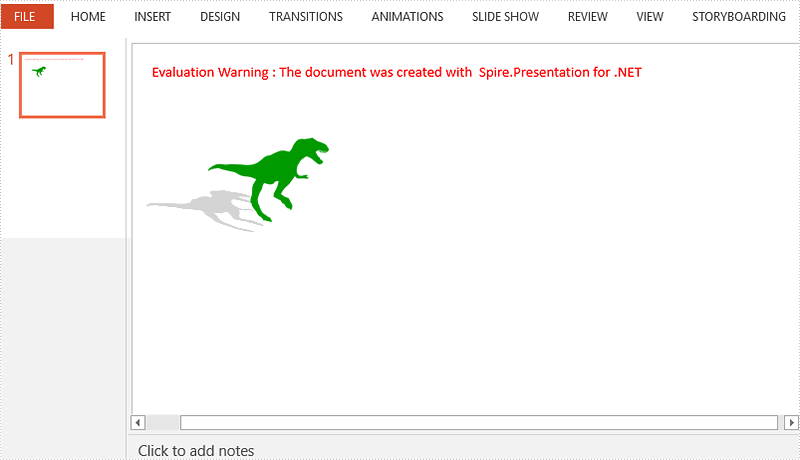Tuesday, 13 March 2018 08:11

### Convert the PDF to word, HTML, SVG, XPS and save them to stream

This article we will demonstrate how to convert the PDF pages to HTML, Word, SVG, XPS, PDF and save them to stream by calling the method PdfDocument.SaveToStream() offered by Spire.PDF. And starts from Spire.PDF version 4.3, it newly supports to convert the defined range of PDF pages and save them to stream.

#### Save the PDF to stream

Step 1: Create a new PdfDocument instance and load the sample document from file.

```PdfDocument pdf = new PdfDocument();
```

Step 2: Save the document to stream.

```MemoryStream ms=new MemoryStream ();
pdf.SaveToStream(ms);
```

#### Save the PDF to stream and defined the file format to HTML, Word, SVG, XPS and PDF

Step 1: Create a new PdfDocument instance and load the sample document from file.

```PdfDocument pdf = new PdfDocument();
```

Step 2: Save the document to stream and use FileFormat format to define the format.

```MemoryStream ms=new MemoryStream ();
pdf.SaveToStream(ms, FileFormat.HTML);
```

#### Convert the defined range of PDF pages to HTML, word, SVG, XPS and save them to stream

Step 1: Create a new PdfDocument instance and load the sample document from file.

```PdfDocument pdf = new PdfDocument();
```

Step 2: Only save some PDF pages to stream by using pdf.SaveToStream(int startIndex, int endIndex, FileFormat format) method; and FileFormat.PDF is not supported.

```pdf.SaveToStream(1, 2, FileFormat.SVG);
```

Full codes of save PDF to stream:

```PdfDocument pdf = new PdfDocument();

MemoryStream ms=new MemoryStream ();
pdf.SaveToStream(ms);
pdf.SaveToStream(ms, FileFormat.HTML);

pdf.SaveToStream(1, 2, FileFormat.SVG);
```
Monday, 12 March 2018 07:55

### Delete Legend and Specific Legend Entries from Excel Chart in C#

A legend is displayed in the chart area by default. However it can be removed from the chart. With Spire.XLS, we can delete the whole legend as well as specific legend entries from Excel chart. This article is going to demonstrate how we can use Spire.XLS to accomplish this function.

Below screenshot shows the Excel chart we used for demonstration: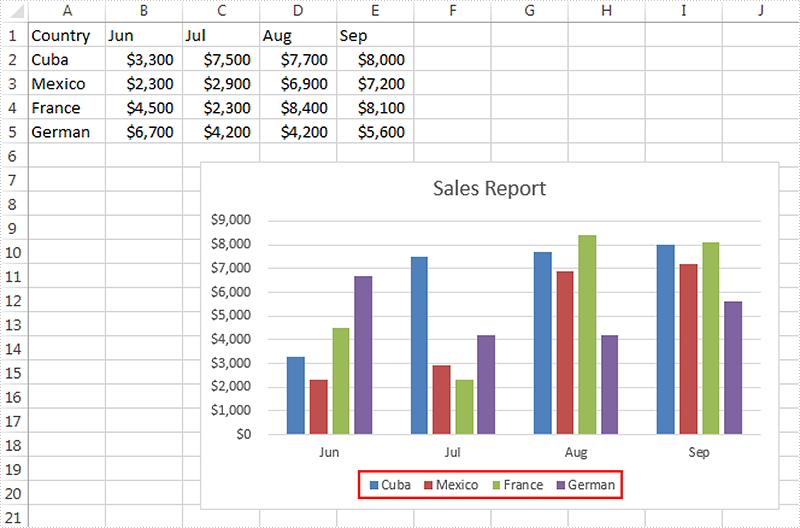Delete the whole legend

```//Create a Workbook instance
Workbook workbook = new Workbook();

//Get the first worksheet
Worksheet sheet = workbook.Worksheets;

//Get the chart
Chart chart = sheet.Charts;

//Delete legend from the chart
chart.Legend.Delete();

//Save the file
workbook.SaveToFile("DeleteLegend.xlsx", ExcelVersion.Version2013);
```

Screenshot: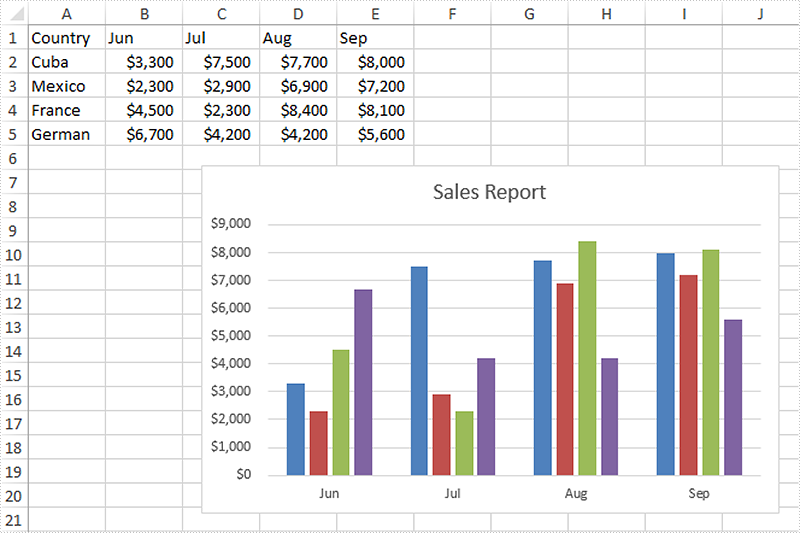Delete specific legend entries

```//Create a Workbook instance
Workbook workbook = new Workbook();

//Get the first worksheet
Worksheet sheet = workbook.Worksheets;

//Get the chart
Chart chart = sheet.Charts;

//Delete the first and the second legend entries from the chart
chart.Legend.LegendEntries.Delete();
chart.Legend.LegendEntries.Delete();

//Save the file
workbook.SaveToFile("DeleteLegendEntries.xlsx", ExcelVersion.Version2013);
```

Screenshot: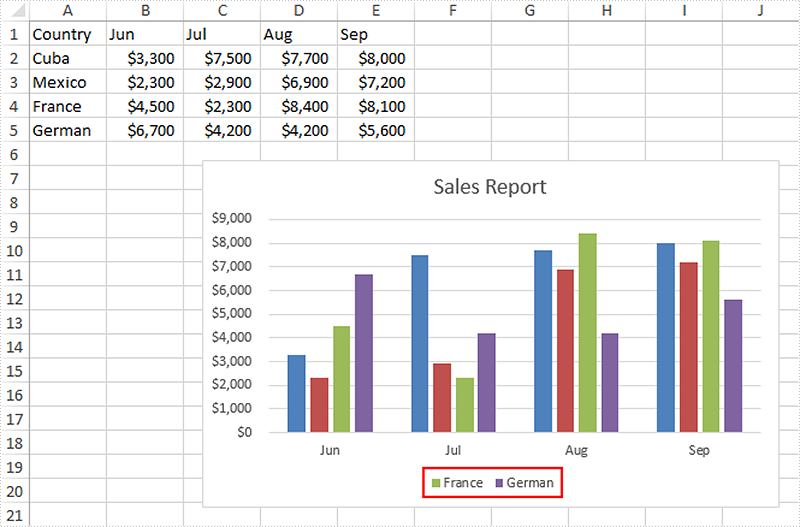Monday, 12 March 2018 07:12

### Create Multi-Level Category Chart in Excel in C#, VB.NET

Multi-level category chart is a chart type that has both main category and subcategory labels. This type of chart is useful when you have figures for items that belong to different categories. In this article, you will learn how to create a multi-level category chart in Excel using Spire.XLS with C# and VB.NET.

Step 1: Create a Workbook instance and get the first worksheet.

```Workbook wb = new Workbook();
Worksheet sheet = wb.Worksheets;
```

Step 2: Write data to cells.

```sheet.Range["A1"].Text = "Main Category";
sheet.Range["A2"].Text = "Fruit";
sheet.Range["A6"].Text = "Vegies";
sheet.Range["B1"].Text = "Sub Category";
sheet.Range["B2"].Text = "Bananas";
sheet.Range["B3"].Text = "Oranges";
sheet.Range["B4"].Text = "Pears";
sheet.Range["B5"].Text = "Grapes";
sheet.Range["B6"].Text = "Carrots";
sheet.Range["B7"].Text = "Potatoes";
sheet.Range["B8"].Text = "Celery";
sheet.Range["B9"].Text = "Onions";
sheet.Range["C1"].Text = "Value";
sheet.Range["C2"].Value = "52";
sheet.Range["C3"].Value = "65";
sheet.Range["C4"].Value = "50";
sheet.Range["C5"].Value = "45";
sheet.Range["C6"].Value = "64";
sheet.Range["C7"].Value = "62";
sheet.Range["C8"].Value = "89";
sheet.Range["C9"].Value = "57";
```

Step 3: Vertically merge cells from A2 to A5, A6 to A9.

```sheet.Range["A2:A5"].Merge();
sheet.Range["A6:A9"].Merge();
```

Step 4: Add a clustered bar chart to worksheet.

```Chart chart = sheet.Charts.Add(ExcelChartType.BarClustered);
chart.ChartTitle = "Value";
chart.PlotArea.Fill.FillType = ShapeFillType.NoFill;
chart.Legend.Delete();
```

Step 5: Set the data source of series data.

```chart.DataRange = sheet.Range["C2:C9"];
chart.SeriesDataFromRange = false;
```

Step 6: Set the data source of category labels.

```ChartSerie serie = chart.Series;
serie.CategoryLabels = sheet.Range["A2:B9"];
```

Step 7: Show multi-level category labels.

```chart.PrimaryCategoryAxis.MultiLevelLable = true;
```

Step 8: Save the file.

```wb.SaveToFile("output.xlsx", ExcelVersion.Version2013);
```

Output: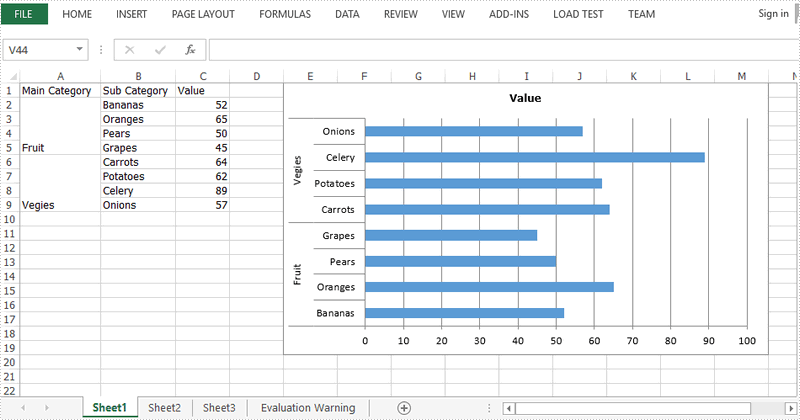Full Code:

[C#]
```Workbook wb = new Workbook();
Worksheet sheet = wb.Worksheets;

sheet.Range["A1"].Text = "Main Category";
sheet.Range["A2"].Text = "Fruit";
sheet.Range["A6"].Text = "Vegies";
sheet.Range["B1"].Text = "Sub Category";
sheet.Range["B2"].Text = "Bananas";
sheet.Range["B3"].Text = "Oranges";
sheet.Range["B4"].Text = "Pears";
sheet.Range["B5"].Text = "Grapes";
sheet.Range["B6"].Text = "Carrots";
sheet.Range["B7"].Text = "Potatoes";
sheet.Range["B8"].Text = "Celery";
sheet.Range["B9"].Text = "Onions";
sheet.Range["C1"].Text = "Value";
sheet.Range["C2"].Value = "52";
sheet.Range["C3"].Value = "65";
sheet.Range["C4"].Value = "50";
sheet.Range["C5"].Value = "45";
sheet.Range["C6"].Value = "64";
sheet.Range["C7"].Value = "62";
sheet.Range["C8"].Value = "89";
sheet.Range["C9"].Value = "57";
sheet.Range["A2:A5"].Merge();
sheet.Range["A6:A9"].Merge();
sheet.AutoFitColumn(1);
sheet.AutoFitColumn(2);

chart.ChartTitle = "Value";
chart.PlotArea.Fill.FillType = ShapeFillType.NoFill;
chart.Legend.Delete();
chart.LeftColumn = 5;
chart.TopRow = 1;
chart.RightColumn = 14;
chart.DataRange = sheet.Range["C2:C9"];
chart.SeriesDataFromRange = false;
ChartSerie serie = chart.Series;
serie.CategoryLabels = sheet.Range["A2:B9"];
chart.PrimaryCategoryAxis.MultiLevelLable = true;
wb.SaveToFile("output.xlsx", ExcelVersion.Version2013);
```
[VB.NET]
```Dim wb As Workbook = New Workbook()
Dim sheet As Worksheet = wb.Worksheets(0)

sheet.Range("A1").Text = "Main Category"
sheet.Range("A2").Text = "Fruit"
sheet.Range("A6").Text = "Vegies"
sheet.Range("B1").Text = "Sub Category"
sheet.Range("B2").Text = "Bananas"
sheet.Range("B3").Text = "Oranges"
sheet.Range("B4").Text = "Pears"
sheet.Range("B5").Text = "Grapes"
sheet.Range("B6").Text = "Carrots"
sheet.Range("B7").Text = "Potatoes"
sheet.Range("B8").Text = "Celery"
sheet.Range("B9").Text = "Onions"
sheet.Range("C1").Text = "Value"
sheet.Range("C2").Value = "52"
sheet.Range("C3").Value = "65"
sheet.Range("C4").Value = "50"
sheet.Range("C5").Value = "45"
sheet.Range("C6").Value = "64"
sheet.Range("C7").Value = "62"
sheet.Range("C8").Value = "89"
sheet.Range("C9").Value = "57"
sheet.Range("A2:A5").Merge()
sheet.Range("A6:A9").Merge()
sheet.AutoFitColumn(1)
sheet.AutoFitColumn(2)

Dim chart As Chart = sheet.Charts.Add(ExcelChartType.BarClustered)
chart.ChartTitle = "Value"
chart.PlotArea.Fill.FillType = ShapeFillType.NoFill
chart.Legend.Delete()
chart.LeftColumn = 5
chart.TopRow = 1
chart.RightColumn = 14
chart.DataRange = sheet.Range("C2:C9")
chart.SeriesDataFromRange = False
Dim serie As ChartSerie =  chart.Series(0)
serie.CategoryLabels = sheet.Range("A2:B9")
chart.PrimaryCategoryAxis.MultiLevelLable = True
wb.SaveToFile("output.xlsx", ExcelVersion.Version2013)
```
Thursday, 08 March 2018 03:46

### How to clone a word document in C#

With Spire.Doc, we can copy the content from one word document to another word document in C#. When we need to generate a large amount of documents from a single document, clone the document will be much easier. The clone method speeds up the generation of the word documents and developers only need one single line of code to get the copy of the word document.

Now we will show the code snippet of how to clone a word document in C#.

Step 1: Create a new instance of Document and load the document from file.

```Document doc = new Document();
```

Step 2: Clone the word document.

```doc.Clone();
```

Step 3: Save the document to file.

```doc.SaveToFile("Cloneword.docx", FileFormat.Docx2010);
```

Effective screenshot of clone the word document: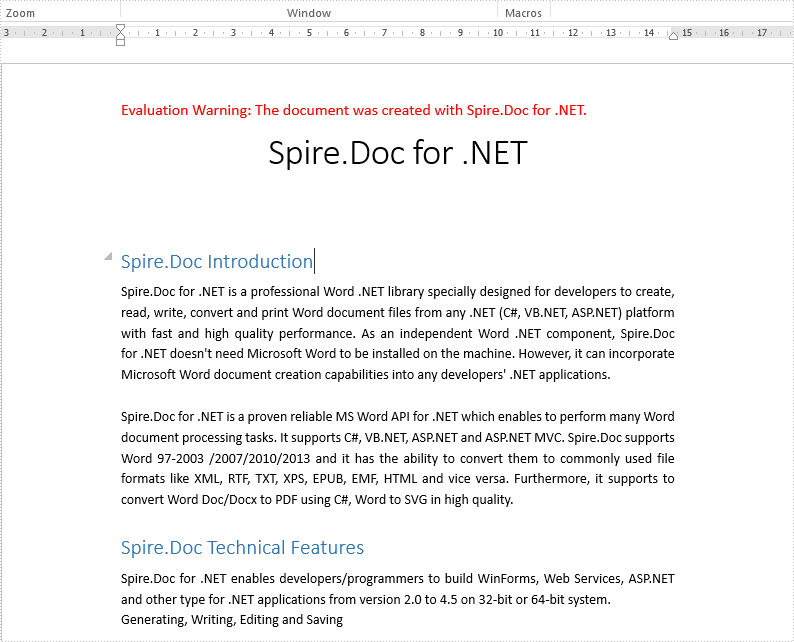Full codes of clone a word document:

```static void Main(string[] args)
{

Document doc = new Document();